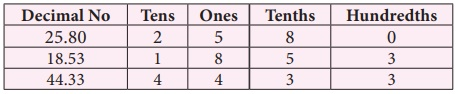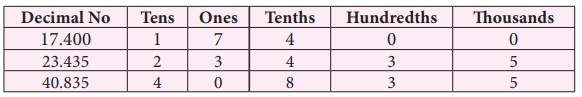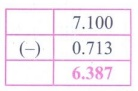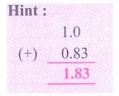Home | | Maths 7th Std | Exercise 1.2 (Addition and Subtraction of Decimal Numbers)

# Exercise 1.2 (Addition and Subtraction of Decimal Numbers)

7th Maths : Term 3 Unit 1 : Number System : Addition and Subtraction of Decimal Numbers : Text Book Back Exercises Questions with Answers, Solution

Exercise 1.2

1. Add by using grid 0.51 + 0.25

Solution:Here 0.51 = 51 / 100 and 0.25 = 25 / 100.

First we shade the region 0.51 and then 0.25.

The sum is the total shaded area.

0.51 + 0.25 = 0.76

2. Add the following by using place value grid.

(i) 25.8 + 18.53

Solution:

25.8 + 18.53.

Using place value grid.Therefore 25.8 + 18.53 = 44.33

(ii) 17.4 + 23.435

Solution:

17.4 + 23.435

Lets use the place value grid.Therefore 17.4 + 23.435 = 40.835

3. Find the value of 0.46 − 0.13 by grid model.

Solution:Here 0.46 = 46 / 100 and 0.13 = 13 / 100

Shading the region 0.46 and then crossing out 0.13 from the shaded area. The left out shaded region without cross marks is the difference.

So 0.46 – 0.13 = 0.33

4. Subtract the following by using place value grid.

(i) 6.567 from 9.231

Solution:

Let as use place value gridTherefore 9.231 – 6.567 = 2.664

(ii) 3.235 from 7

Solution:

Let as use place value grid.Therefore 7 – 3.235 = 3.765

5. Simplify: 23.5 − 27.89 + 35.4 − 17.

Solution:

23.5 – 27.89 + 35.4 – 17 = 14.016. Sulaiman bought 3.350 kg of Potato, 2.250 kg of Tomato and some Onions. If the weight of the total items are 10.250 kg, then find the weight of Onions?

Solution:

Weight of Potato = 3.350 kg

Weight of Tomato = 2.250 kg

Total weight of Potato and Tomato = (3.350 + 2.250 kg)

= 5.600 kg

Weight of potato, tomato and onions = 10.250

Weight of potato and tomato = 5.600

Weight of onions = (10.250 – 5.600) kg = 4.650 kg

Weight of onions = 4.650 kg

7. What should be subtracted from 7.1 to get 0.713?

Solution:

To get the number to be subtracted

We have 7.1–0.713 = 6.387The number to be subtracted = 6.387

8. How much is 35.6 km less than 53.7 km?

Solution:

To get the answer we must subtract 53.7km – 35.6 km = 18.1 kmSo 35.6 km is 18.1 km less than 53.7 km.

9. Akilan purchased a geometry box for ₹ 25.75, a pencil for ₹ 3.75 and a pen for ₹ 17.90. He gave ₹ 50 to the shopkeeper. What amount did he get back?

Solution:Cost of geometry box = ₹ 25.75 (+)

Cost of Pencil box = ₹ 3.75

Cost of a pen = ₹ 17.90

Amount to be paid = ₹ 47.40

Amount paid = ₹ 50.00 (–)

Amount to be paid = ₹ 47.40

Amount to get back = ₹ 2.60

Amount to get back = ₹ 2.60

10. Find the perimeter of an equilateral triangle with a side measuring 3.8 cm.

Solution:

Perimeter of an equilateral triangle = (Side + Side + Side) Sq. units.

Given side = 3.8

∴ Perimeter = 3.8 + 3.8 + 3.8Perimeter of the triangle = 11.4 cm

Objective type questions

11. 1.0 + 0.83=?

(i) 0.17

(ii) 0.71

(iii) 1.83

(iv) 1.3812. 7.0 – 2.83 = ?

(i) 3.47

(ii) 4.17

(iii) 7.34

(iv) 4.7313. Subtract 1.35 from 3.51

(i) 6.21

(ii) 4.86

(iii) 8.64

(iv) 2.1614. Sum of two decimals is 4.78. If one decimal is 3.21, then the other one is

(i)1.57

(ii) 1.75

(iii)1.59

(iv) 1.5815. The difference of two decimals is 86.58 and one of the decimal is 42.31. Find the other one

(i) 128.89

(ii) 128.69

(iii) 128.36

(iv) 128.39Exercise 1.2

1.Ans 0.76

2. (i)(ii)3.4. (i)(ii)5. 14.01

6. 4.650

7. 6.387

8. 18.1

9. 2.60

10. 11.4 cm

Objective type questions

11. (iii) 1.83

12. (ii) 4.17

13. (iv) 2.16

14. (i) 1.57

15. (i) 128.89

Tags : Questions with Answers, Solution | Number System | Term 3 Chapter 1 | 7th Maths , 7th Maths : Term 3 Unit 1 : Number System
Study Material, Lecturing Notes, Assignment, Reference, Wiki description explanation, brief detail
7th Maths : Term 3 Unit 1 : Number System : Exercise 1.2 (Addition and Subtraction of Decimal Numbers) | Questions with Answers, Solution | Number System | Term 3 Chapter 1 | 7th Maths